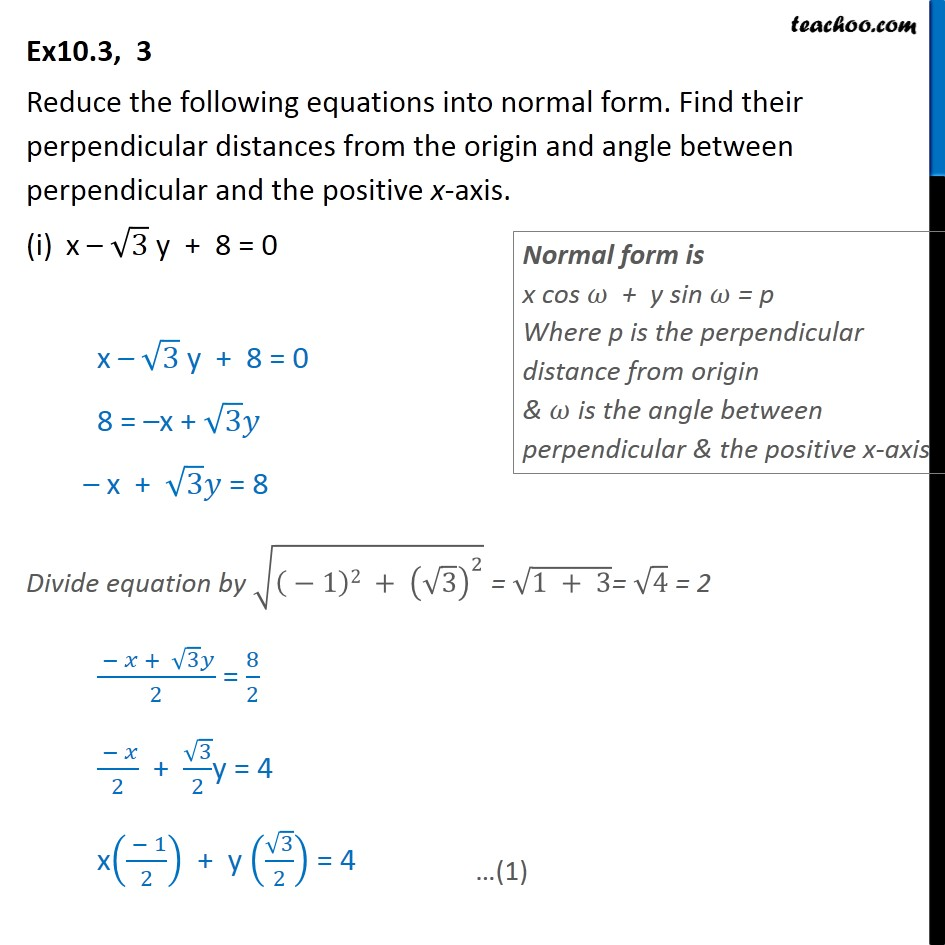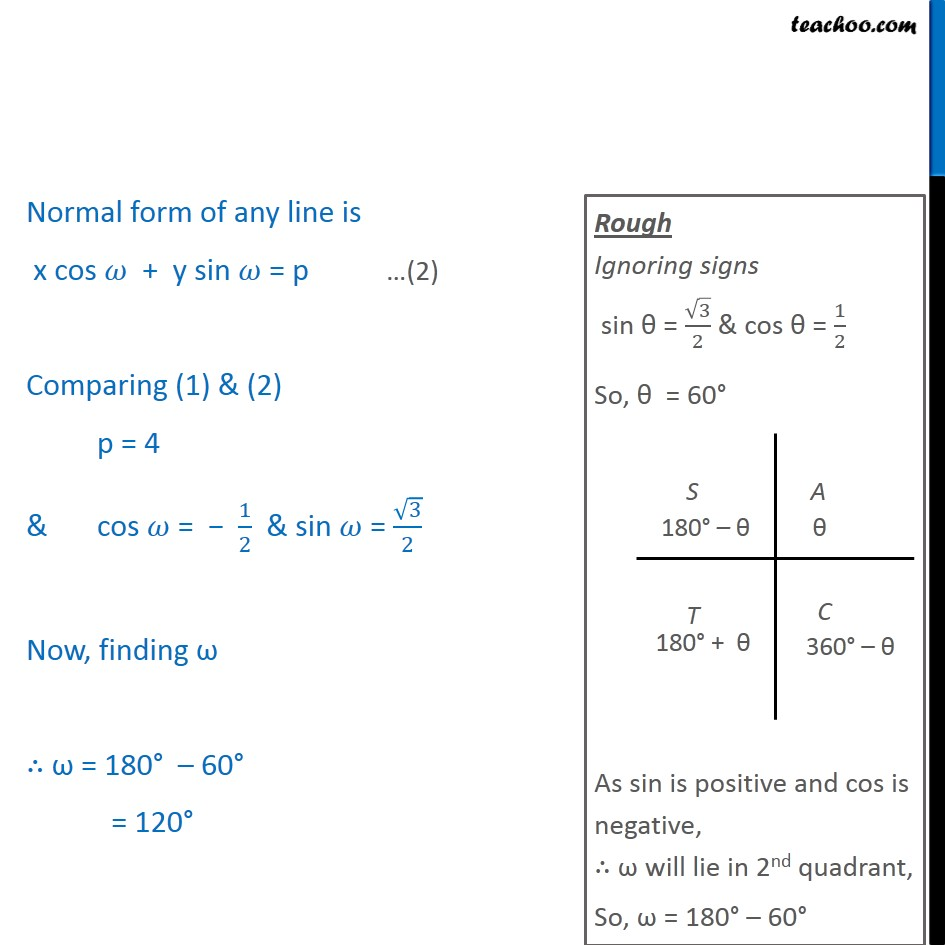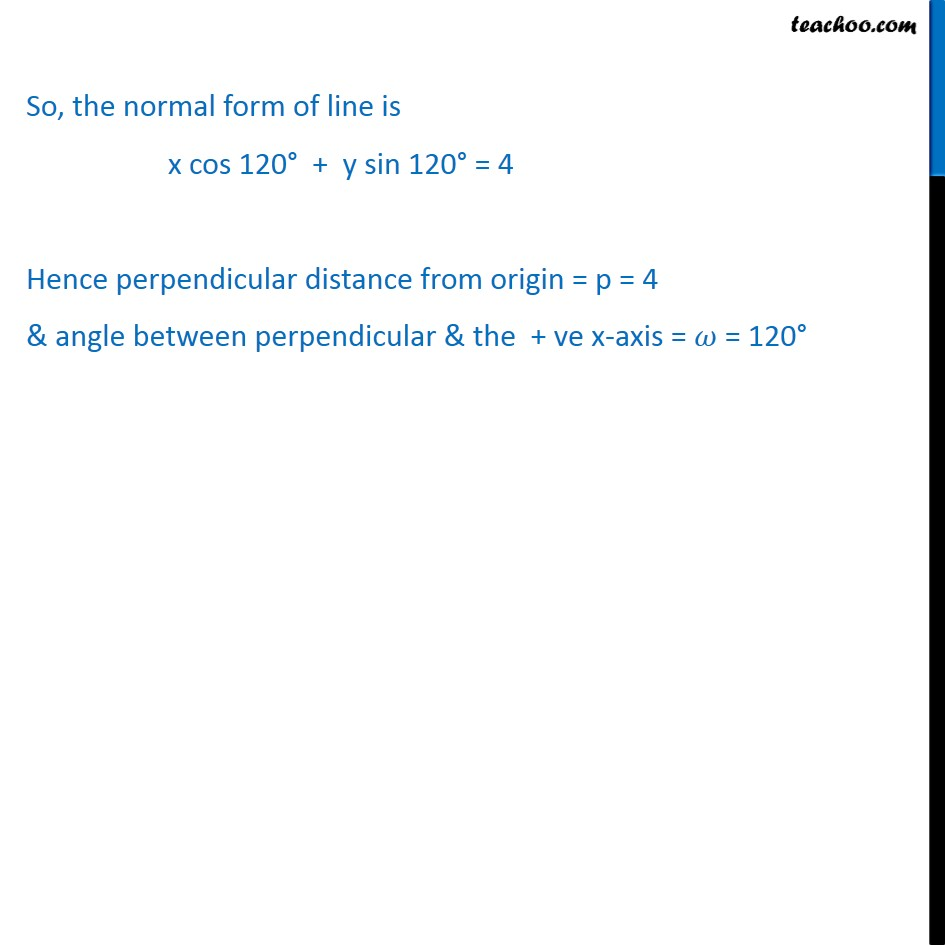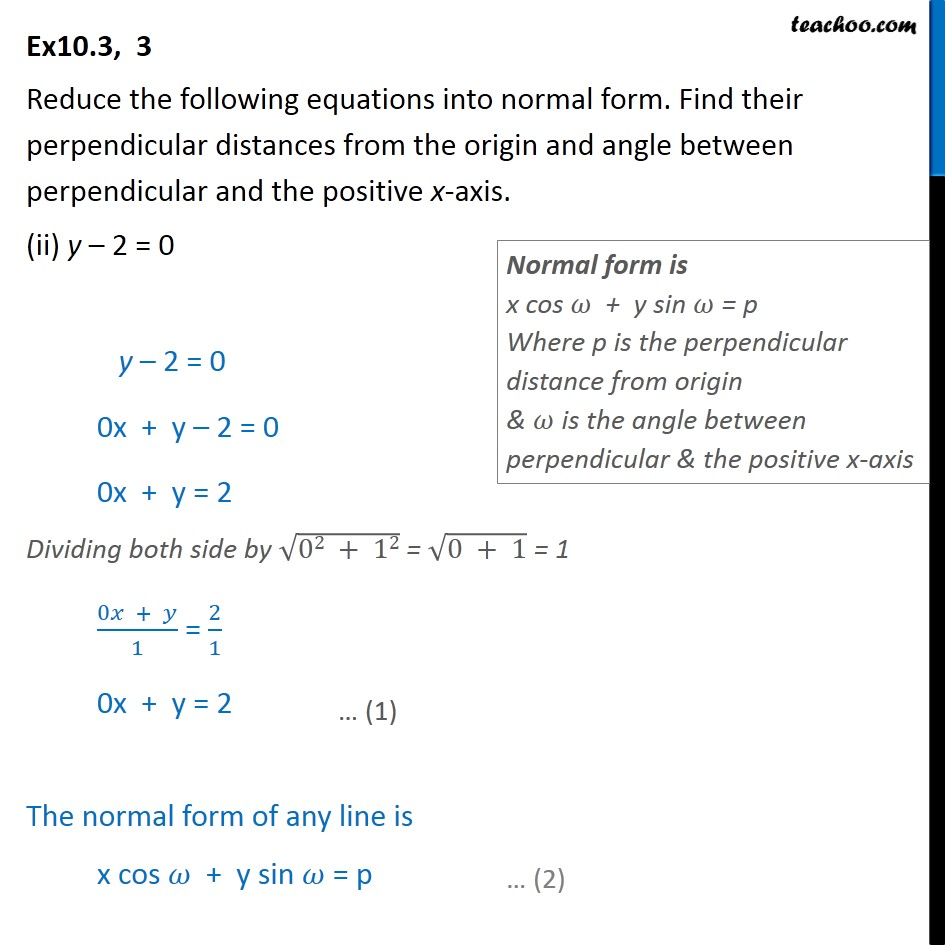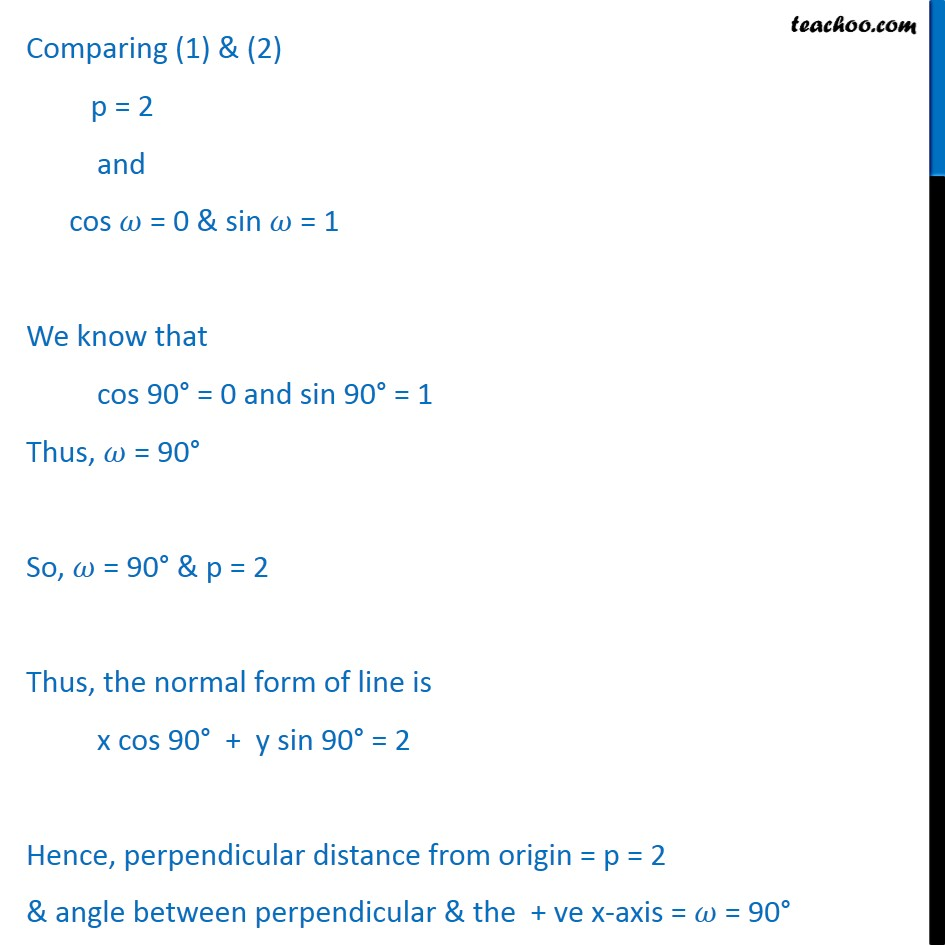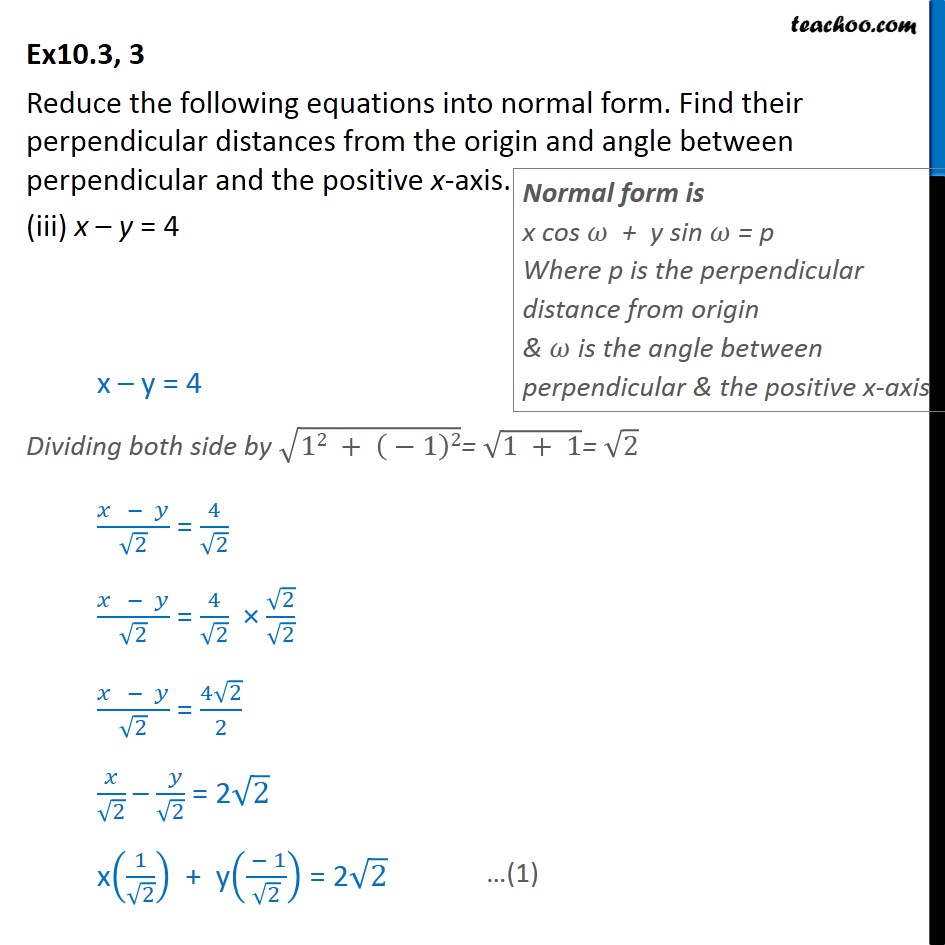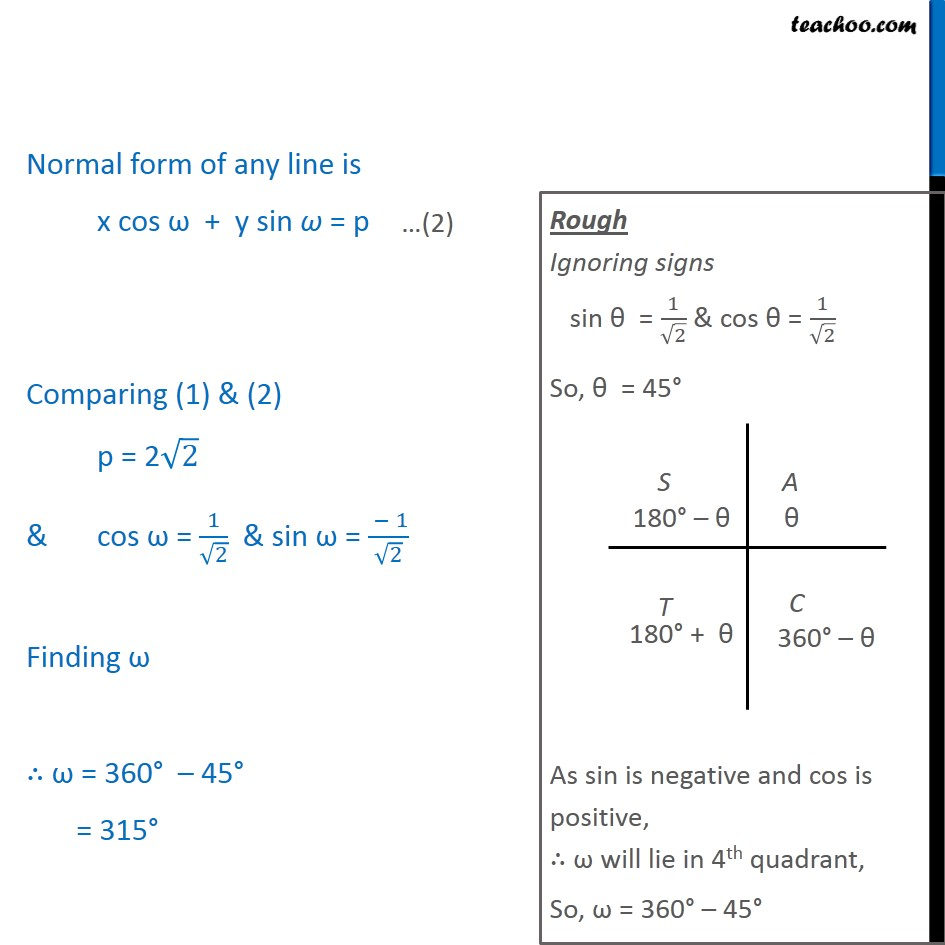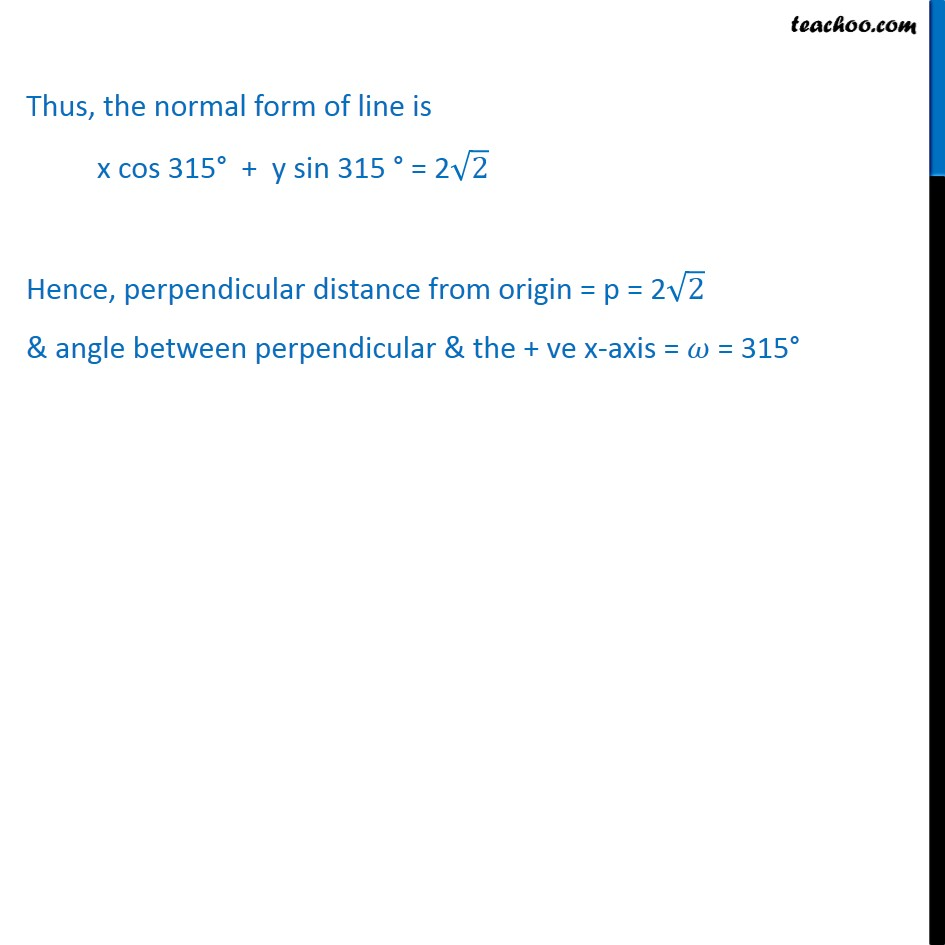Subscribe to our Youtube Channel - https://you.tube/teachoo

1. Chapter 10 Class 11 Straight Lines
2. Serial order wise
3. Ex 10.3

Transcript

Ex10.3, 3 Reduce the following equations into normal form. Find their perpendicular distances from the origin and angle between perpendicular and the positive x-axis. x 3 y + 8 = 0 x 3 y + 8 = 0 8 = x + 3 x + 3 = 8 Divide equation by (( 1)2 + ( 3)^2 ) = (1 + 3)= 4 = 2 ( + 3 )/2 = 8/2 ( )/2 + 3/2y = 4 x(( 1)/2) + y ( 3/2) = 4 Normal form of any line is x cos + y sin = p Comparing (1) & (2) p = 4 & cos = 1/2 & sin = 3/2 Now, finding = 180 60 = 120 So, the normal form of line is x cos 120 + y sin 120 = 4 Hence perpendicular distance from origin = p = 4 & angle between perpendicular & the + ve x-axis = = 120 Ex10.3, 3 Reduce the following equations into normal form. Find their perpendicular distances from the origin and angle between perpendicular and the positive x-axis. (ii) y 2 = 0 y 2 = 0 0x + y 2 = 0 0x + y = 2 Dividing both side by (02 + 12) = (0 + 1) = 1 (0 + )/1 = 2/1 0x + y = 2 The normal form of any line is x cos + y sin = p Comparing (1) & (2) p = 2 and cos = 0 & sin = 1 We know that cos 90 = 0 and sin 90 = 1 Thus, = 90 So, = 90 & p = 2 Thus, the normal form of line is x cos 90 + y sin 90 = 2 Hence, perpendicular distance from origin = p = 2 & angle between perpendicular & the + ve x-axis = = 90 Ex10.3, 3 Reduce the following equations into normal form. Find their perpendicular distances from the origin and angle between perpendicular and the positive x-axis. (iii) x y = 4 x y = 4 Dividing both side by (12 + ( 1)2)= (1 + 1)= 2 ( )/ 2 = 4/ 2 ( )/ 2 = 4/ 2 2/ 2 ( )/ 2 = (4 2)/2 / 2 ( )/ 2 = 2 2 x(1/ 2) + y(( 1)/ 2) = 2 2 Normal form of any line is x cos + y sin = p Comparing (1) & (2) p = 2 2 & cos = 1/ 2 & sin = ( 1)/ 2 Finding = 360 45 = 315 Thus, the normal form of line is x cos 315 + y sin 315 = 2 2 Hence, perpendicular distance from origin = p = 2 2 & angle between perpendicular & the + ve x-axis = = 315

Ex 10.3